# How to Use a Multiplication Chart

Use this guide for a straightforward approach to understanding multiplication charts, an essential tool to hone your child’s multiplication skills.Author
Michelle GriczikaPublished
November 8, 2023# How to Use a Multiplication Chart

Use this guide for a straightforward approach to understanding multiplication charts, an essential tool to hone your child’s multiplication skills.Author
Michelle GriczikaPublished
Nov 8, 2023# How to Use a Multiplication Chart

Use this guide for a straightforward approach to understanding multiplication charts, an essential tool to hone your child’s multiplication skills.Author
Michelle GriczikaPublished
November 8, 2023Key takeaways

• Multiplication charts are practical, hands-on tools that promote children’s understanding and memorization of their times tables.
• Reading a multiplication chart is as simple as spotting your desired numbers and identifying where they intersect.

Is your child starting with multiplication? If so, a multiplication chart might just be their new best friend. This friendly chart is nothing more than a grid, but it’s a grid that shows multiplication facts in a clear, easy-to-understand way. It’s a practical tool that presents multiplication facts in a format that’s easy to follow, aiding children in their understanding and memorization of multiplication principles. You might say, a multiplication chart takes the mystery out of multiplication.

## What is a Multiplication Chart?A multiplication chart, often called a times table or multiplication table, is a grid that illustrates the result of multiplying pairs of numbers. The chart is designed as a grid that displays columns of consecutive numbers across the top and rows of consecutive numbers to the left. You can find the product of two numbers (aka factors) by tracing where they intersect on the grid.

## How to Read a Multiplication ChartReading a multiplication chart or timetable chart might seem overwhelming, but it’s pretty simple. Let’s break it down:

1. Locate the numbers: The first step to using a multiplication chart is identifying the numbers you want to multiply. These will be listed down the left side (rows) and across the top (columns) of the chart.

2. Find the intersection: Once you’ve identified the two numbers you’re multiplying, follow the row of the first number and the column of the second number. The point where the row and column intersect on the chart is the result of the multiplication.

So, if you were trying to solve 7 x 8 on a multiplication chart, you would find the number 7 on the left-hand side and follow that row across to the right until you come to the eighth column. Where the two numbers meet is where you’ll see the answer – 56.

The process gets even easier with more practice. Remember, like any other skill, mastering multiplication takes time, so be patient with your child and offer plenty of encouragement as they learn.

## Free Printable Multiplication ChartsPracticing multiplication is the secret to getting better at it. That’s why we’ve got a variety of multiplication chart printables ready for you to use. Whether your child is starting with multiplication or ready to tackle bigger challenges, a printable multiplication chart here is right for them.

#### Multiplication Chart 1-12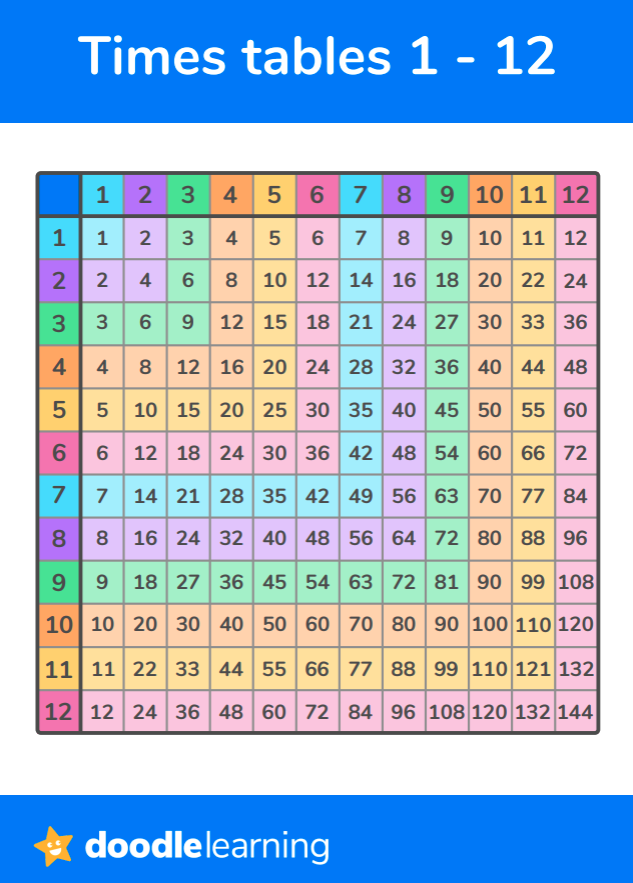#### Multiplication Chart 1-20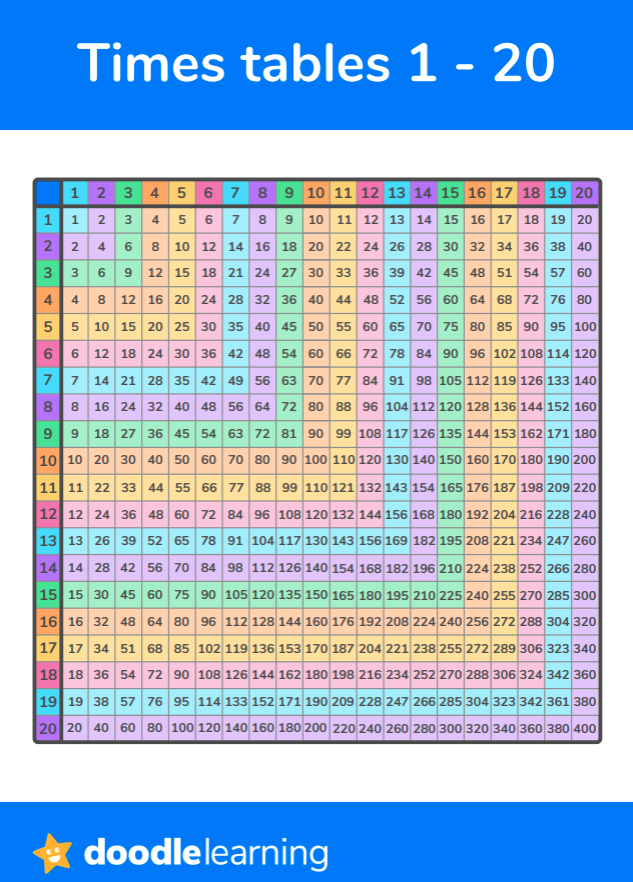#### Multiplication Chart 1-10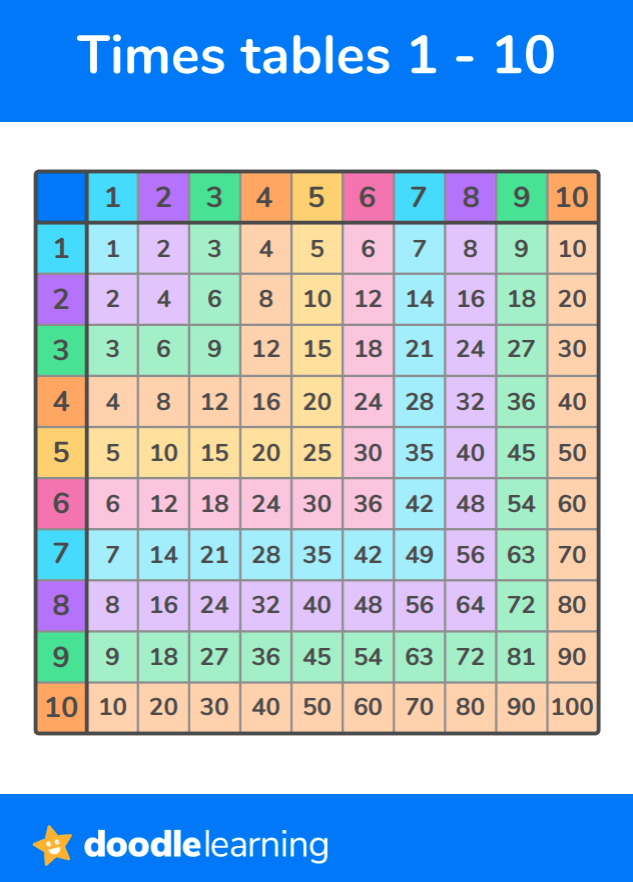#### Blank Multiplication Charts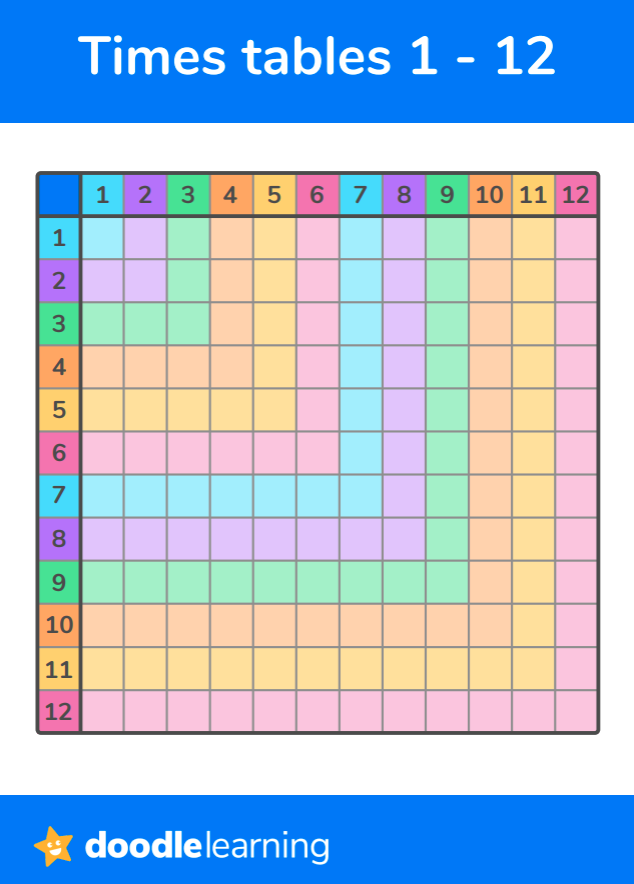## Multiplication Property Practice Problems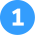Apply the associative property of multiplication to simplify these problems:

1. (2 * 3) * 4 = ?
2. 5 * (3 * 4) = ?
3. (7 * 2) * 3 = ?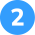Use the commutative property of multiplication to rewrite and solve these problems:

1. 7 * 6 = ? Can you rewrite and solve this?
2. 8 * 3 = ? What is it when rewritten?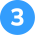Use the distributive property of multiplication to simplify these problems:

1. 3 * (4 + 2) = ?
2. 5 * (6 + 3) = ?
3. 2 * (5 + 7) = ?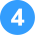Use the identity property of multiplication to solve these problems:

1. 9 * 1 =
2. 1 * 6 =
3. 5 * 1 =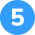Apply the zero property of multiplication to solve these problems:

1. 8 * 0 = ?
2. 0 * 5 = ?
3. 3 * 0 = ?Associative Property of Multiplication

1. (2 * 3) * 4 = 24
2. 5 * (3 * 4) = 60
3. (7 * 2) * 3 = 42Commutative Property of Multiplication

1. 7 * 6 = 42; 6 * 7 = 42
2. 8 * 3 = 24; 3 * 8 = 24
3. 2 * 9 = 36; 9 * 2 = 18Distributive Property of Multiplication

1. 3 * (4 + 2) = 18
2. 5 * (6 + 3) = 45
3. 2 * (5 + 7) = 24Identity Property of Multiplication

1. 9 * 1 = 9
2. 1 * 6 = 6
3. 5 * 1 = 5Zero Property of Multiplication

1. 8 * 0 = 0
2. 0 * 5 = 0
3. 3 * 0 = 0

## FAQs About Times Tables ChartsA multiplication table for kids is a tool that displays the results of multiplying two numbers together. It’s arranged in a grid format, visually representing the concept of multiplication, and helps kids better understand and memorize these basic math facts.

We use a multiplication chart or time table chart because it’s an excellent visual aid that simplifies the learning of multiplication. It provides a quick reference for multiplication facts and aids in understanding the patterns and relationships between numbers. This ultimately helps children to develop their number sense and mathematical reasoning skills.

Reading a multiplication chart involves finding the values you wish to multiply along the top row and the first column, then following the row and column of these numbers until they intersect. The value at the intersection point is the product of the multiplication.

Creating a multiplication chart starts by writing the numbers 1 to 10 or 1 to 12 (depending on the chart size you want to make) across the top row and down the first column. Then, fill in each cell in the chart by multiplying the number from its row by the number from its column. So, for example, the cell at the intersection of row 3 and column 4 would contain the number 12 since 3 times 4 equals 12. Repeat this process for all the cells in the chart.Title 1

Title 2

Title 3

## Related Posts

Title 1Title 2Title 3### In summary...Remember, practicing consistently is vital to mastering these properties. For additional practice, you can check out this math help app. It’s an excellent tool for extra help and provides more exercises.

Lesson credits

Michelle Griczika

Michelle Griczika is a seasoned educator and experienced freelance writer. Her years teaching first and fifth grades coupled with her double certification in elementary and early childhood education lend depth to her understanding of diverse learning stages. Michelle enjoys running in her free time and undertaking home projects.

Michelle Griczika

Michelle Griczika is a seasoned educator and experienced freelance writer. Her years teaching first and fifth grades coupled with her double certification in elementary and early childhood education lend depth to her understanding of diverse learning stages. Michelle enjoys running in her free time and undertaking home projects.# Are you a parent, teacher or student?

Are you a parent or teacher?

## Hi there!

Book a chat with our team